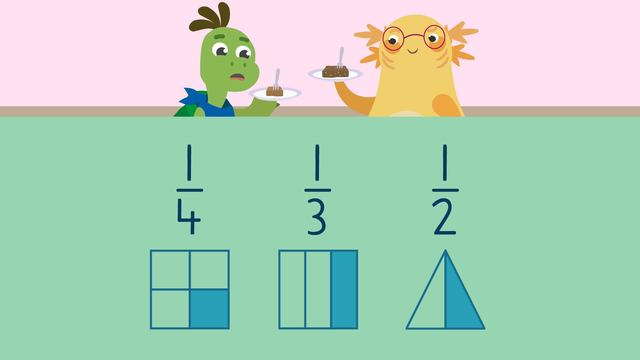# Unit fractionsRate this video

Ø 5.0 / 2 ratings

The authorTeam Digital
Unit fractions
CCSS.MATH.CONTENT.3.NF.A.1

## Information about the videoUnit fractions

### In This Video

Axel and Tank are a fudge that sells fudge in unit fraction sizes. They want to know, what is a unit fraction? They learn about unit fractions, and finally settle down for some delicious fudge!

### What is a Unit Fraction in Math?

You may ask yourself, what is a unit fraction in 3rd grade math? Well, a unit fraction is a fraction that has a numerator of one. As long as the numerator is one, it is a unit fraction.

### What is a Unit Fraction Example

A unit fraction has a numerator of one, such as one fourth, one third, and one half.If a fraction does not have a numerator of one, it is not a unit fraction. For example, three fourths would not be a unit fraction since the numerator is three, and not one.### Summary of Unit Fractions

Remember, a unit fraction is a fraction with a numerator of one. Below you will find worksheets that will help you learn what is a unit fraction, 3rd grade.

### TranscriptUnit fractions

Axel and Tank are at a fudge store that only sells fudge in unit fractions. There are only two pieces left. "I'll take the one fourth piece, it will be bigger because it has a four in it!" "Fine, I'll take the one third piece, it's probably smaller because it has a three in it." Let's see who gets the bigger piece by learning about unit fractions. A fraction represents a part of a whole. The bottom number tells you how many parts make up the whole, and is called a denominator. The top number is the numerator, and represents how many parts we have. Unit Fractions are fractions that have a numerator of one, like one-fourth, one-third, and one-half. We can represent unit fractions with fraction bars or shapes. Let's see if you can recognize unit fractions! Here is the first one. What fraction do we see? It has four parts in all, so the denominator is two. Three parts are shaded in, so the numerator is three. Is three fourths a unit fraction? It is not a unit fraction, because it does not have one as it's numerator! Now look at this one. What fraction do we see? It has two parts in all, so the denominator is two. One part is shaded in, so the numerator is one. Is one half a unit fraction? One half is a unit fraction, because it has a one for the numerator! As Axel and Tank wait for their fudge let's look at their order! Tank ordered one fourth, and Axel ordered one third! If you look at the denominator and the size of each piece of fudge, you might notice something special about fractions! What do you notice? When comparing fraction bars, objects, or shapes, that are the same size, the greater the number of parts a fraction has, the smaller each part is! This is because we are dividing the whole into more equal parts! Even though four is a greater number than three, each part in the one fourth fraction bar is smaller than each part in the one thirds fraction bar because there are more equal parts in one quarter than one third. Let's review unit fractions! Remember, a unit fraction must have a numerator of one, like these fractions! A greater denominator means each part of the unit fraction is smaller, because we are dividing the whole into more equal parts. "Hey Tank, do you realize what we just learned?" "What do you mean?" "Well, you ordered the one fourth unit fraction piece of fudge, thinking it was going to be bigger than my one third piece of fudge." "Oh no! The bigger denominator means I actually have the smaller piece!"